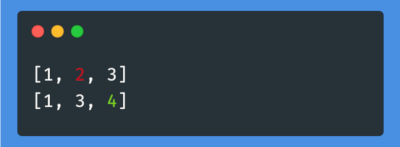## seqdiff

Diff between two sequences

### 8 releases

 0.3.0 Mar 31, 2021 Sep 15, 2020 Jun 21, 2020

#1014 in Algorithms

Used in 2 crates (via tokenizations)

19KB
402 lines

# SeqDiff - Diff between two sequences for Rust and Python

Functions to get correspondence between two sequences like `diff`, based on Myers' algorithm.# Usage

## Python

### `diff`

``````def diff(
a: Sequence[S], b: Sequence[T], *, key: Optional[Callable[[S, T], bool]] = None
) -> Tuple[List[Optional[int]], List[Optional[int]]]: ...
``````

Returns the correspondence between two sequences `a` and `b`. `key` is a comparison function (if needed).

``````import seqdiff

a = [1, 2, 3]
b = [1, 3, 4]
a2b, b2a = seqdiff.diff(a, b)
assert all(a[i] == b[j] for i, j in enumerate(a2b) if j is not None)
assert all(a[j] == b[i] for i, j in enumerate(b2a) if j is not None)
print(a2b)
print(b2a)
``````
``````[0, None, 1]
[0, 2, None]
``````

### `print_diff`

``````def print_diff(
a: Sequence[S], b: Sequence[T], *, key: Optional[Callable[[S, T], bool]] = None
): ...
``````

Pretty print the diff between two sequences `a` and `b`.
`key` is a comparison function (if needed).

``````import seqdiff

a = [1, 2, 3]
b = [1, 3, 4]
a2b, b2a = seqdiff.print_diff(a, b)
``````

The return value is a pair of tuples. The first tuple contains the index where the item from the first sequence appears in the 2nd sequence or `None` if the item doesn't appear in the 2nd sequence. The 2nd tuple is the same but listing the corresponding indexes for the 2nd sequence in the first sequence.## Rust

``````use seqdiff;

let (a2b, b2a) = seqdiff::diff(&[1, 2, 3], &[1, 3]);
assert_eq!(a2b, vec![Some(0), None, Some(1)]);
assert_eq!(b2a, vec![Some(0), Some(2)]);
``````